# Automatic Image Quality Assessment in Python

Image quality is a notion that highly depends on observers. Generally,
it is linked to the conditions in which it is viewed; therefore, it is a highly subjective topic. Image quality assessment aims to quantitatively represent the human perception of quality. These metrics are commonly used to analyze the performance of algorithms in different fields of computer vision like image compression, image transmission, and image processing .

Image quality assessment (IQA) is mainly divided into two areas of research (1) reference-based evaluation and (2) no-reference evaluation. The main difference is that reference-based methods depend on a high-quality image as a source to evaluate the difference between images. An example of reference-based evaluations is the Structural Similarity Index (SSIM) .

### No-reference Image Quality Assessment

No-reference image quality assessment does not require a base image to evaluate image quality, the only information that the algorithm receives is a distorted image whose quality is being assessed.

Blind methods are mostly comprised of two steps. The first step calculates features that describe the image’s structure and the second step finds the patterns among the features to human opinion. TID2008 is a famous database created following a methodology that describes how to measure human opinion scores from referenced images . It is widely used to compare the performance of IQA algorithms.

### Blind/referenceless image spatial quality evaluator (BRISQUE)

In this section, we will code step by step how the BRISQUE method in python. You can find the complete notebook here.

BRISQUE  is a model that only uses the image pixels to calculate features (other methods are based on image transformation to other spaces like wavelet or DCT). It is demonstrated to be highly efficient as it does not need any transformation to calculate its features.

BRISQUE relies on spatial Natural Scene Statistics (NSS) model of locally normalized luminance coefficients in the spatial domain, as well as the model for pairwise products of these coefficients.

#### Natural Scene Statistics in the Spatial Domain

Given an image, we need to compute the locally normalized luminescence via local mean subtraction and divide it by the local deviation. A constant is added to avoid zero divisions.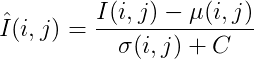*Hint: If I(i, j) domain is [0, 255] then C=1 if the domain is [0, 1] then C=1/255.

To calculate the locally normalized luminescence, also known as mean subtracted contrast normalized (MSCN) coefficients, we have to calculate the local mean. Here, w is a Gaussian kernel of size (K, L).The way that the author displays the local mean could be a little bit confusing but it is calculated by just applying a Gaussian filter to the image.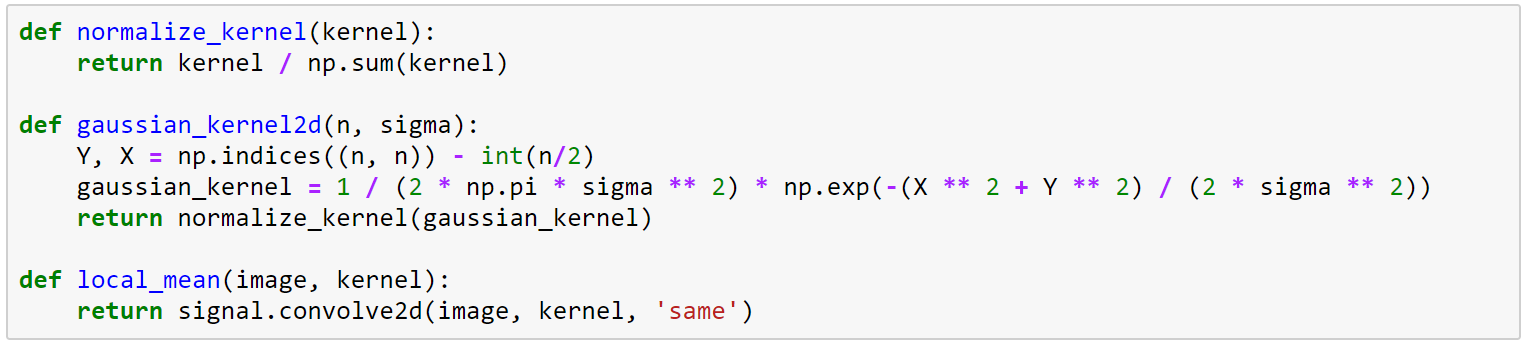Then, we calculate the local deviation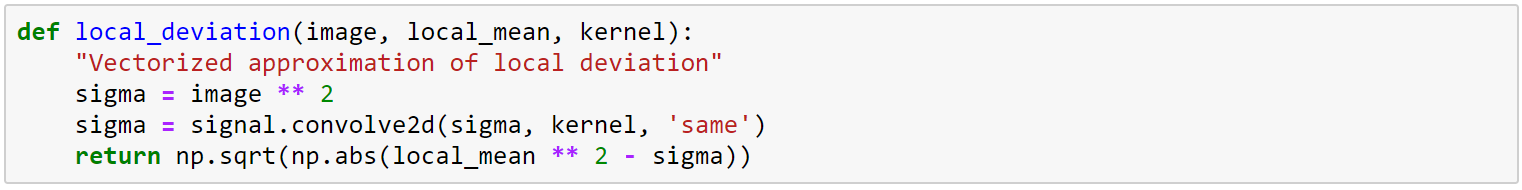Finally, we calculate the MSCN coefficients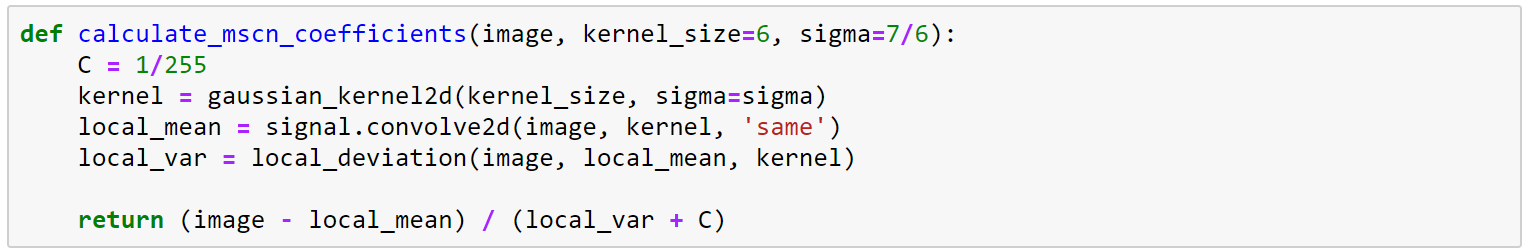The author found that the MSCN coefficients are distributed as a Generalized Gaussian Distribution (GGD) for a broader spectrum of the distorted image. The GGD density function is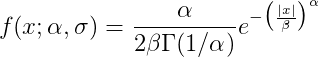whereand Г is the gamma function. The parameter α controls the shape and σ² the variance.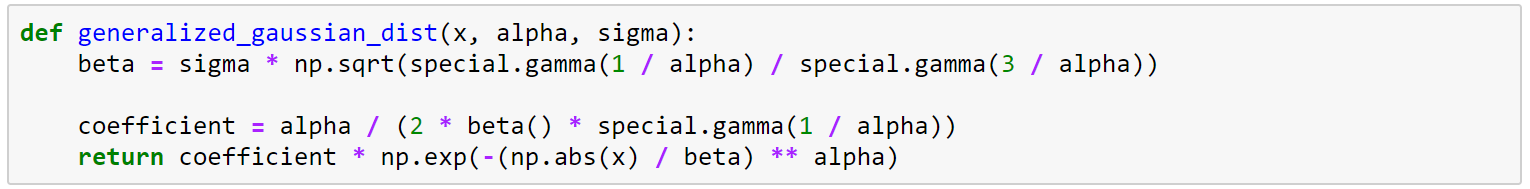#### Pairwise products of neighboring MSCN coefficients

The signs of adjacent coefficients also exhibit a regular structure, which gets disturbed in the presence of distortion. The author proposes the model of pairwise products of neighboring MSCN coefficients along four directions (1) horizontal H, (2) vertical V, (3) main-diagonal D1 and (4) secondary-diagonal D2.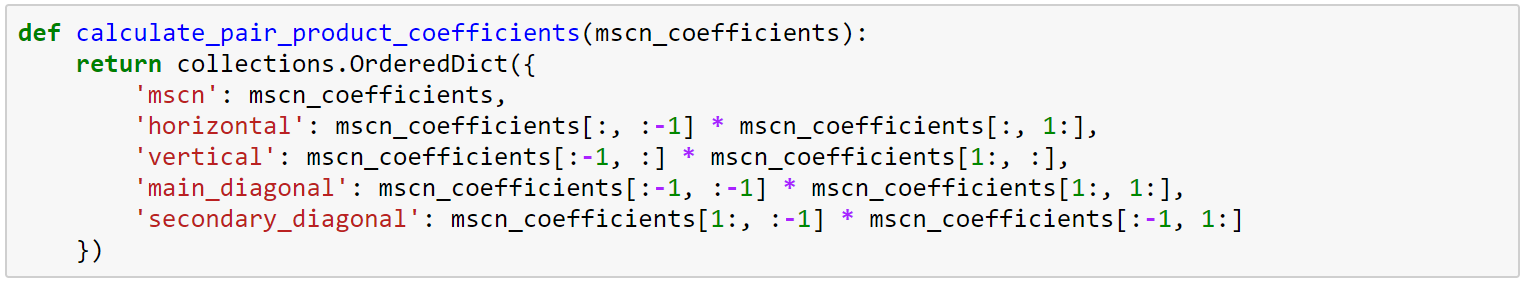Also, he mentions that the GGD does not provide a good fit to the empirical histograms of coefficient products. Thus, instead of fitting these coefficients to GGD, they propose to fit an Asymmetric Generalized Gaussian Distribution (AGGD) model . The AGGD density function iswhereand side can be either r or l. Another parameter that is not reflected in the previous formula is the mean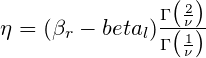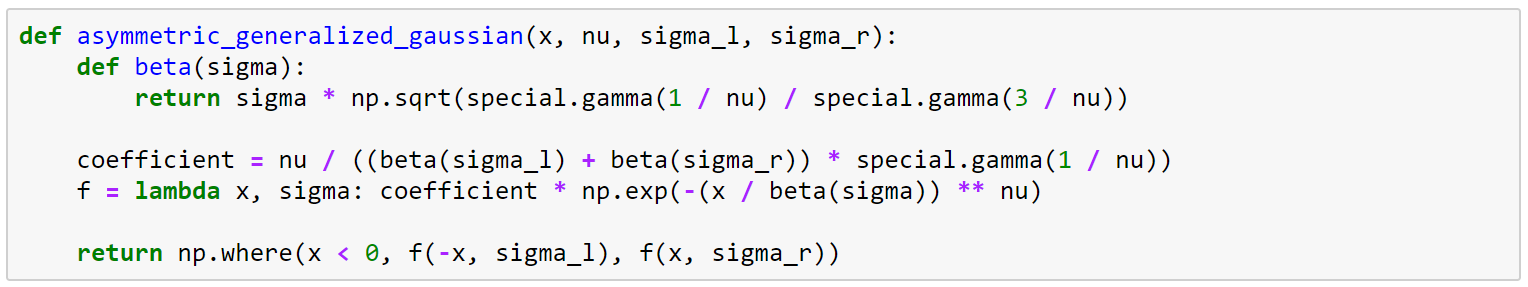#### Fitting Asymmetric Generalized Gaussian Distribution

The methodology to fit an Asymmetric Generalized Gaussian Distribution is described in . In summary, the algorithm steps are:

1. Calculate γ where Nₗ is the number of negative samples and Nᵣ is the number of positive samples.2. Calculate r hat.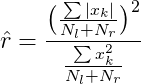3. Calculate R hat using γ and r hat estimations.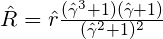4. Estimate α using the approximation of the inverse generalized Gaussian ratio.5. Estimate left and right scale parameters.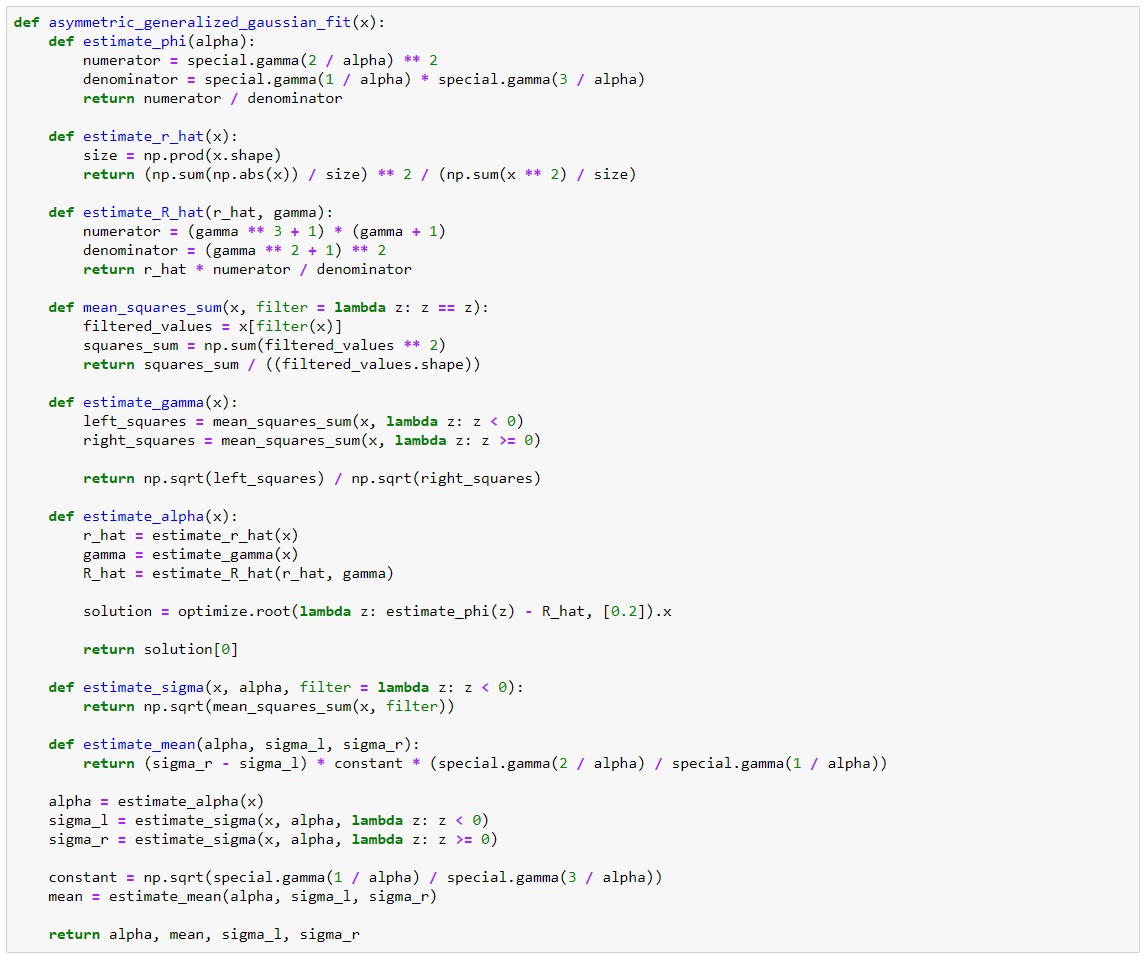#### Calculate BRISQUE features

The features needed to calculate the image quality are the result of fitting the MSCN coefficients and shifted products to the Generalized Gaussian Distributions. First, we need to fit the MSCN coefficients to the GDD, then the pairwise products to the AGGD. A summary of the features is the following: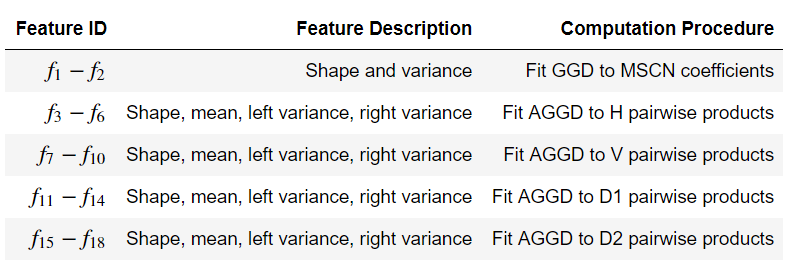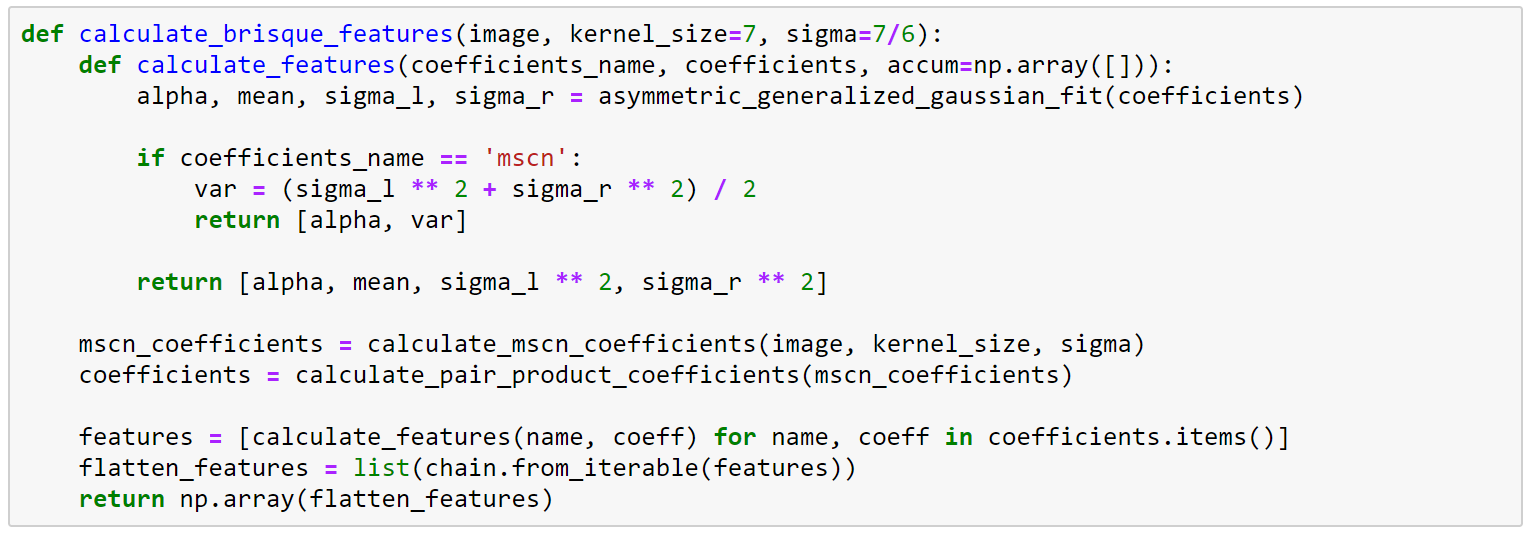### Hands-on

After creating all the functions needed to calculate the BRISQUE features, we can estimate the image quality for a given image. In , they use an image that comes from the Kodak dataset , so we will use it here too.

#### Auxiliary Functions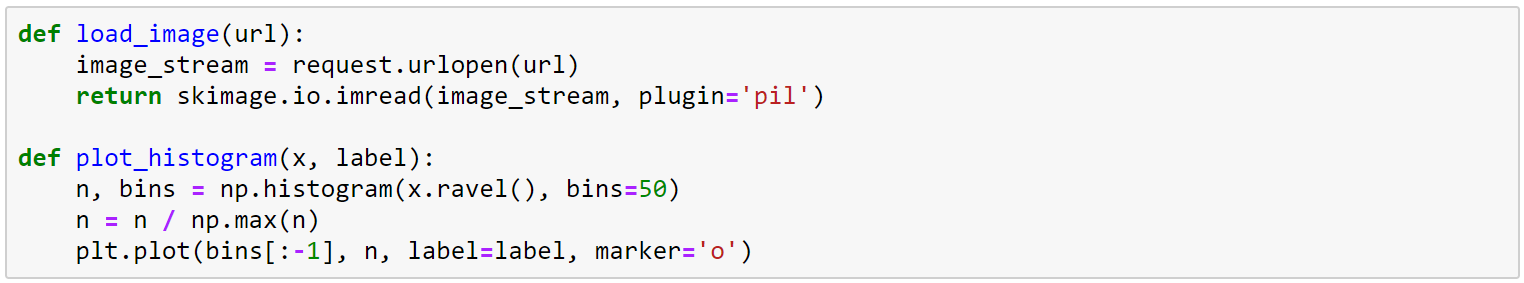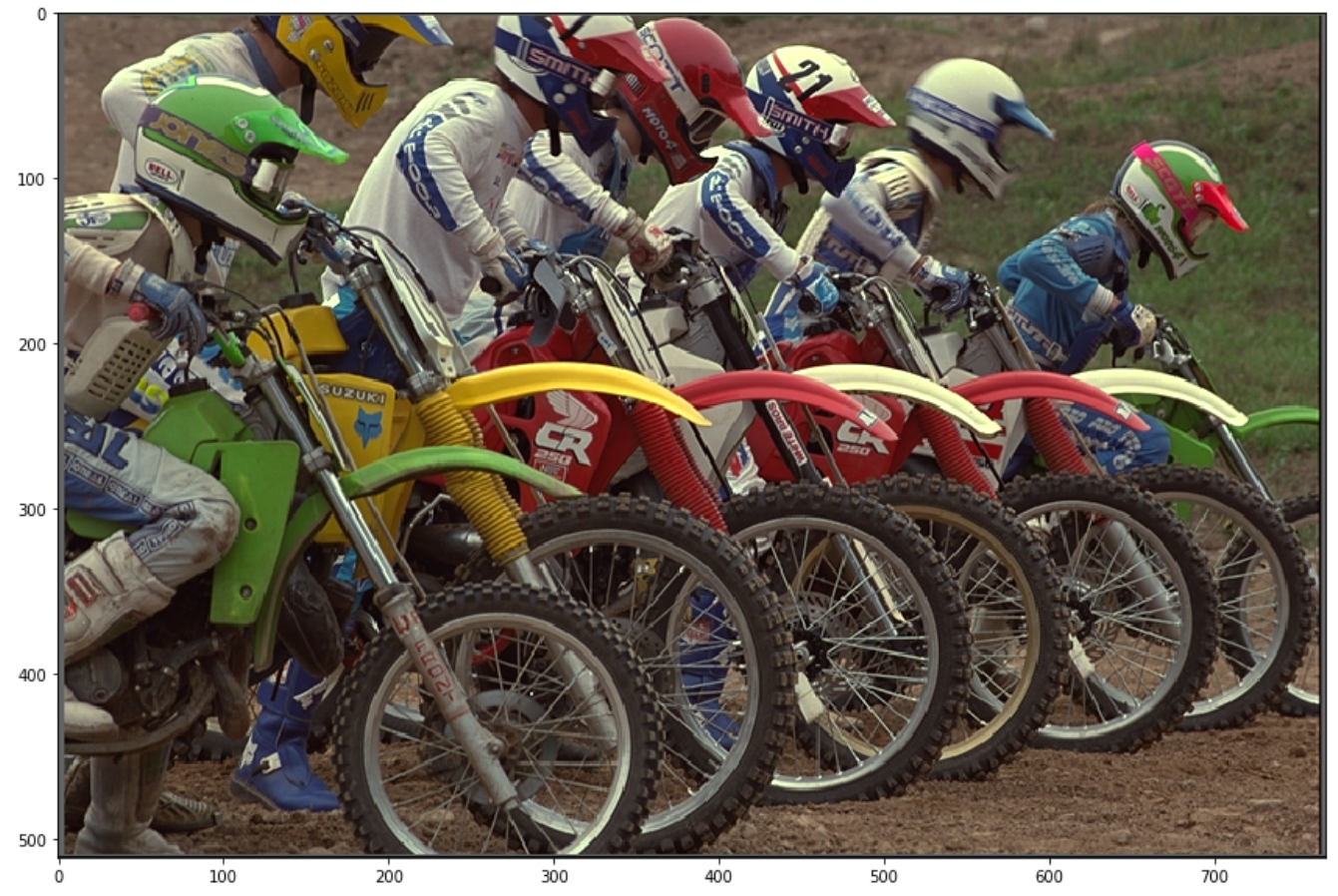#### 2. Calculate Coefficients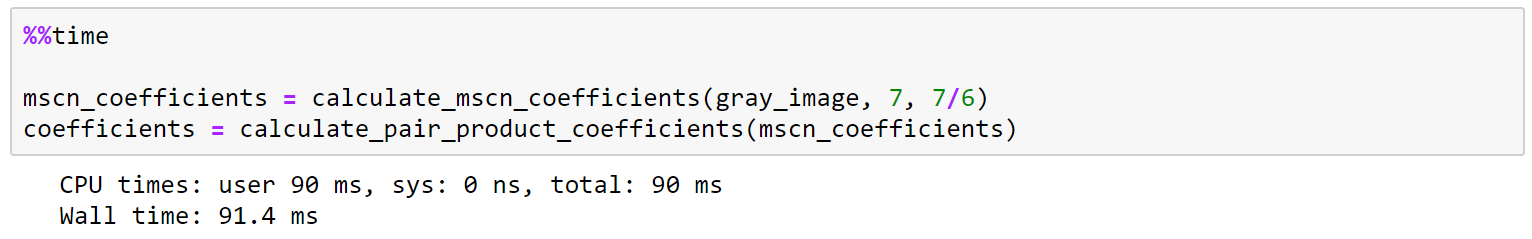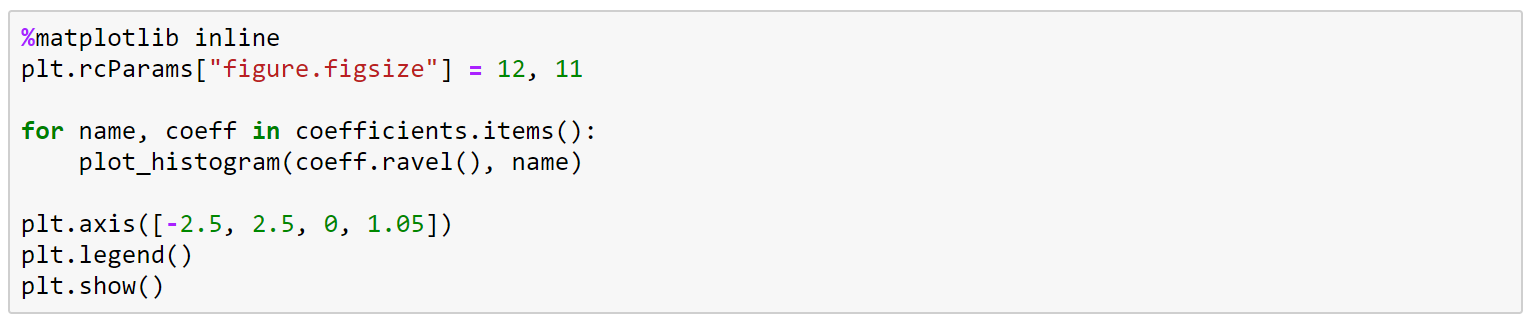After calculating the MSCN coefficients and the pairwise products, we can verify that the distributions are in fact different.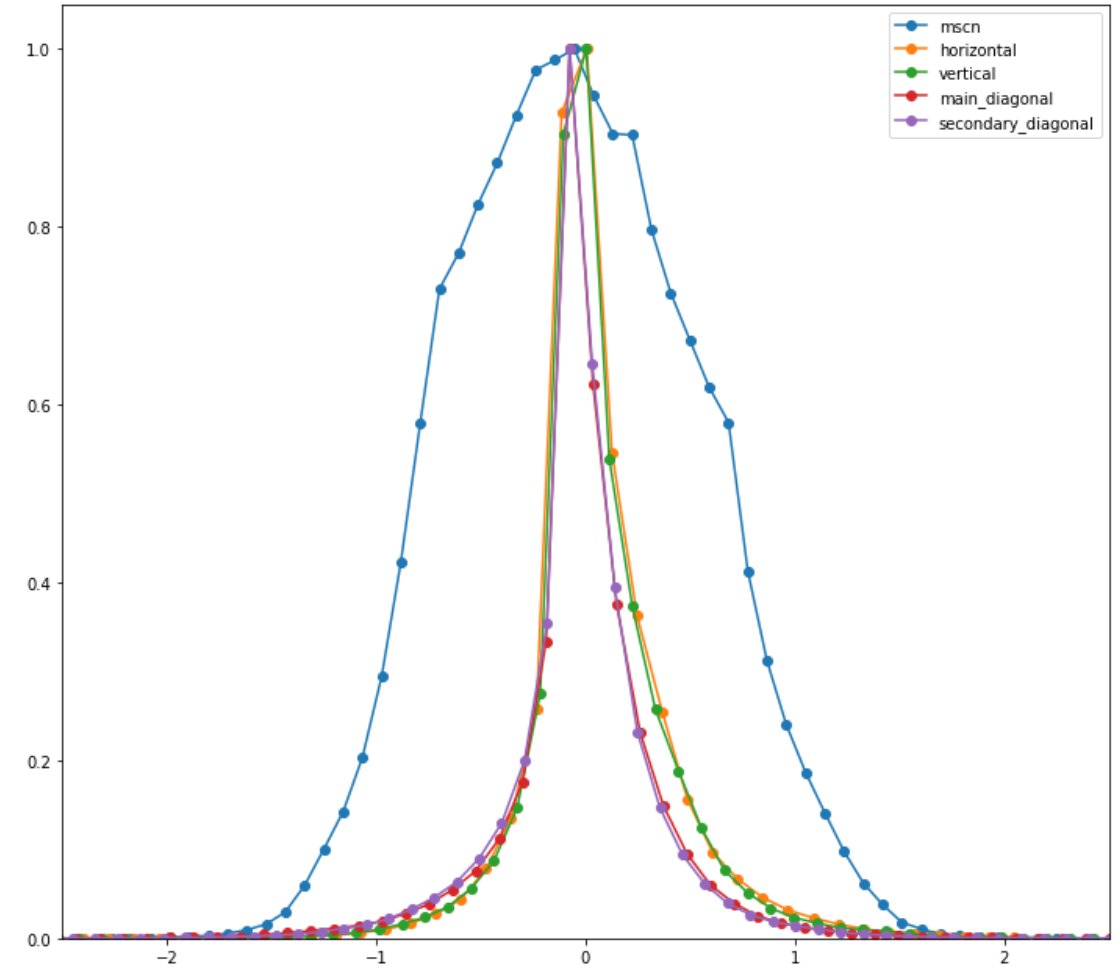#### 3. Fit Coefficients to Generalized Gaussian Distributions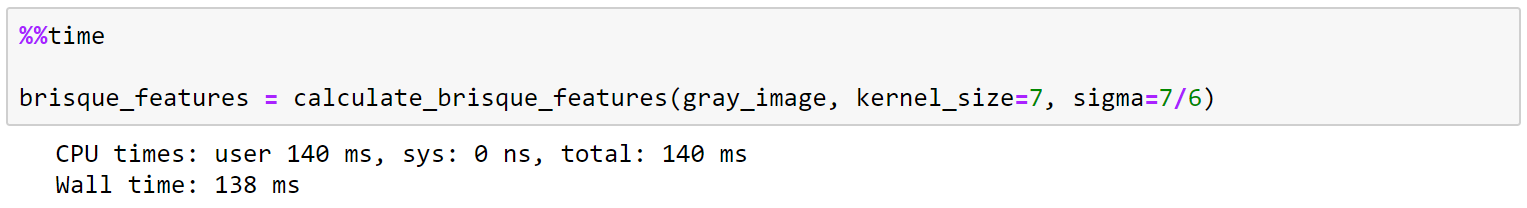#### 4. Resize image and Calculate BRISQUE Features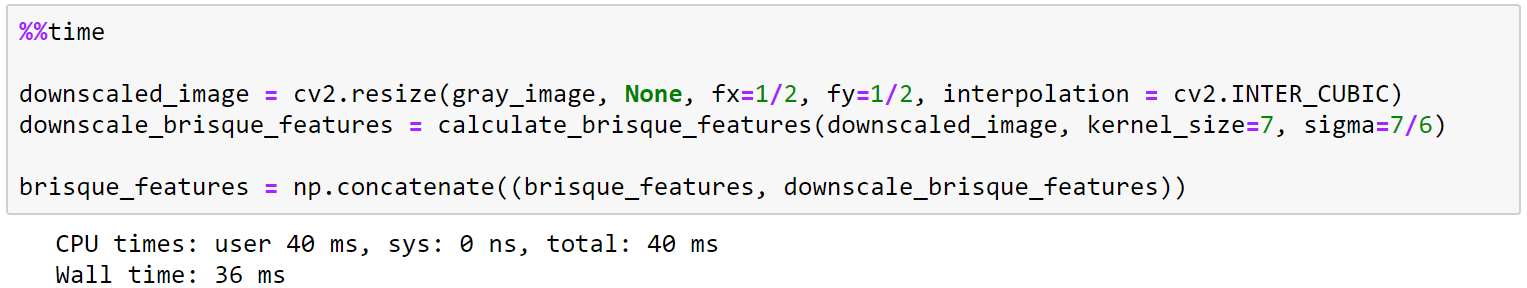#### 5. Scale Features and Feed the SVR

The author provides a pre-trained SVR model to calculate the quality assessment. However, in order to have good results, we need to scale the features to [-1, 1]. For the latter, we need the same parameters as the author used to scale the features vector.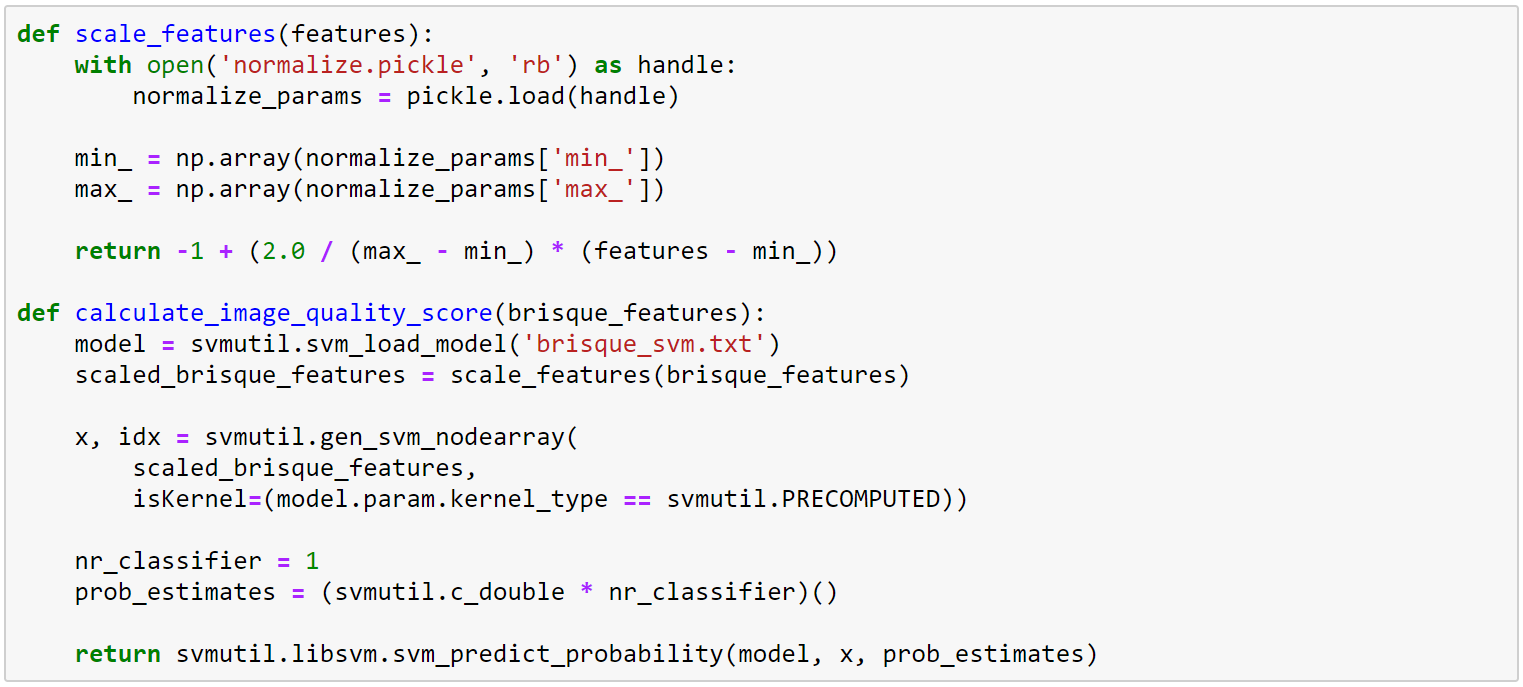The scale used to represent image quality goes from 0 to 100. An image quality of 100 means that the image’s quality is very bad. In the case of the analyzed image, we get that it is a good quality image.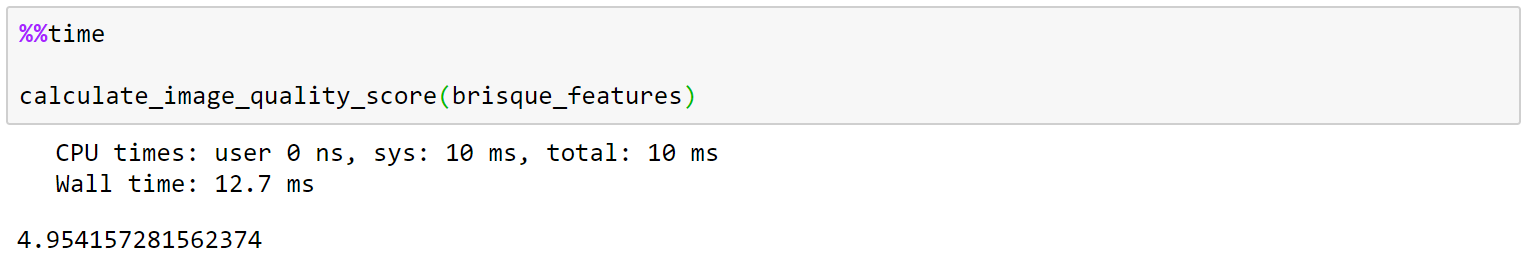### Conclusion

This method was tested with the TID2008 database and performs well; even compared with referenced IQA methods. I would like to check the performance of other machine learning algorithms like XGBoost, LightGBM, for the pattern recognition step.

### Python Notebook

https://github.com/ocampor/notebooks/blob/master/notebooks/image/quality/brisque.ipynb

### References

 Maître, H. (2017). From Photon to pixel: the digital camera handbook. John Wiley & Sons.

 Wang, Z., Bovik, A. C., Sheikh, H. R., & Simoncelli, E. P. (2004). Image quality assessment: from error visibility to structural similarity. IEEE transactions on image processing, 13(4), 600–612.

 Ponomarenko, N., Lukin, V., Zelensky, A., Egiazarian, K., Carli, M., & Battisti, F. (2009). TID2008-a database for evaluation of full-reference visual quality assessment metrics. Advances of Modern Radioelectronics, 10(4), 30–45.

 Mittal, A., Moorthy, A. K., & Bovik, A. C. (2012). No-reference image quality assessment in the spatial domain. IEEE Transactions on Image Processing, 21(12), 4695–4708.

 Lasmar, N. E., Stitou, Y., & Berthoumieu, Y. (2009). Multiscale skewed heavy-tailed model for texture analysis. Proceedings — International Conference on Image Processing, ICIP, (1), 2281–2284.